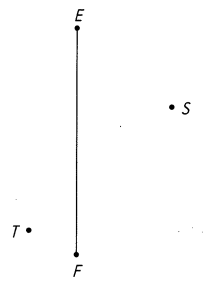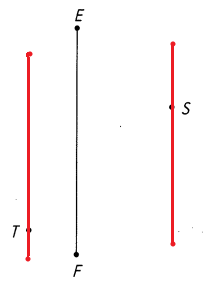# Math in Focus Grade 4 Chapter 10 Practice 2 Answer Key Drawing Parallel Line Segments

This handy Math in Focus Grade 4 Workbook Answer Key Chapter 10 Practice 2 Drawing Parallel Line Segments detailed solutions for the textbook questions.

## Math in Focus Grade 4 Chapter 10 Practice 2 Answer Key Drawing Parallel Line Segments

Use a drawing triangle to draw perpendicular line segments.

Example

Draw a line segment parallel to $$\overline{A B}$$.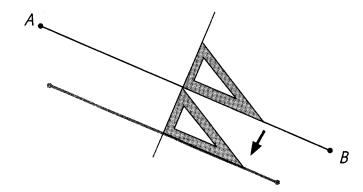Question 1.
Draw a pair of parallel line segments.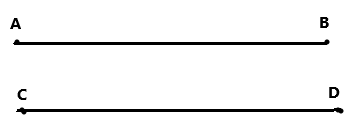Drawn a pair of parallel line segments.

Use a drawing triangle and a straightedge to draw parallel line segments.

Question 2.
Draw a line segment parallel to $$\overline{C D}$$ through point M.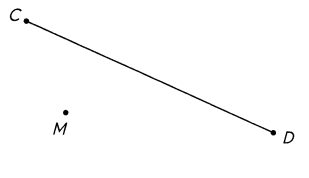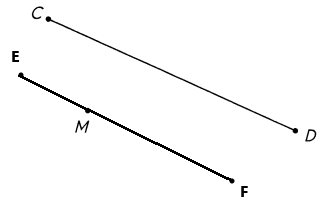Draw a line segment parallel to $$\overline{E F}$$ through point T. Then, draw another line segment parallel to $$\overline{E F}$$ through point S.